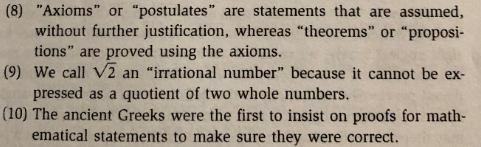(8) "Axioms" or "postulates" are statements that are assumed,without further justification, whereas "theorems" or "proposi-tions" are proved using the axioms.pressed as a quotient of two whole numbers.ematical statements to make sure they were correct.(9) We call V2 an "irrational number" because it cannot be ex-(10) The ancient Greeks were the first to insist on proofs for math-

Question

College Geometry (Proof):
State whether the following statements are correct or not:
Give a short explanation for each.help_outlineImage Transcriptionclose(8) "Axioms" or "postulates" are statements that are assumed, without further justification, whereas "theorems" or "proposi- tions" are proved using the axioms. pressed as a quotient of two whole numbers. ematical statements to make sure they were correct. (9) We call V2 an "irrational number" because it cannot be ex- (10) The ancient Greeks were the first to insist on proofs for math- fullscreen
Step 1

The question comprises multiple parts. Here, I will be answering the first question only, that is number 8. Kindly repost the question specifying the question you need help with.

Step 2

The given statement is:

“Axioms” or “postulates” are statements that are assumed, without further justification, whereas “theorems” or “propositions” are proved using the axioms.

The g...

Want to see the full answer?

See Solution

Want to see this answer and more?

Our solutions are written by experts, many with advanced degrees, and available 24/7

See Solution
Tagged in

Math